﻿ 基于图像处理的硬币分类算法研究 Research of Coin Classification Algorithm Based on Image Processing

Journal of Image and Signal Processing
Vol. 07  No. 04 ( 2018 ), Article ID: 27190 , 9 pages
10.12677/JISP.2018.74026

Research of Coin Classification Algorithm Based on Image Processing

Manli Wang1,2, Rongzu He1, Cuixia Ma3, Hong’an Wang3

1School of Electrical and Information Engineering, Beijing University of Civil Engineering and Architecture, Beijing

2Beijing Key Laboratory of Intelligent Processing for Building Big Data, Beijing

3Institute of Software Chinese Academy of Sciences, Beijing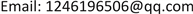Received: Sep. 28th, 2018; accepted: Oct. 13th, 2018; published: Oct. 20th, 2018ABSTRACT

Currently, aiming at the problem that the cost of the coin identification system in the coin trading of the automatic selling machine is too high, this paper designs an algorithm with low cost and high recognition rate. The algorithm mainly takes several common methods of image processing to complete the detection and identification of coins. The core method is to save the information of the circular coin into a two-dimensional array by means of polar coordinates, and then perform feature extraction and feature comparison, and finally perform recognition and determination. The algorithm can effectively avoid the influence of various factors such as the color, size, pattern and illumination of the coin. After a large number of experiments, the correct rate of the algorithm is stable and recognition speed is high, so it has certain application value.

Keywords:Image Processing, Coin Recognition, Polar Coordinates, Matrix

1北京建筑大学电气与信息工程学院，北京

2建筑大数据智能处理方法研究北京市重点实验室，北京

3中国科学院软件研究所，北京1. 引言

2. 硬币识别流程设计

2.1. 硬币特征分析

2.2. 硬币识别流程

3. 硬币图像预处理

3.1. 彩色图像灰度处理

3.2. 图像滤波

4. 边缘检测和图像定位

4.1. 边缘检测

$Gx=\left[\begin{array}{ccc}-1& 0& +1\\ -2& 0& +2\\ -1& 0& +1\end{array}\right]*A$ (1)

$Gy=\left[\begin{array}{ccc}-1& -2& -1\\ 0& 0& 0\\ +1& +2& +1\end{array}\right]*A$ (2)

$G=\sqrt{G{x}^{2}+G{y}^{2}}$ (3)Figure 1. Coin identification flow chart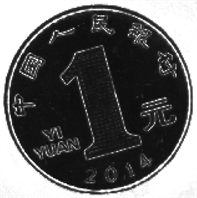Figure 2. Grayscale processed image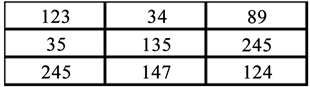Figure 3. Image pixel to be filtered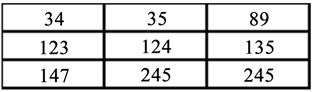Figure 4. Median filtered result image

$\Theta =\mathrm{arctan}\left(\frac{Gx}{Gy}\right)$ (4)

4.2. 边界定位

$r=\left(a+b\right)/2$ (5)

$e=\text{4π}S/{C}^{2}$ (6)

$C=2\text{π}r$ (7)

$S=\text{π}{r}^{\text{2}}$ (8)

5. 硬币识别

5.1. 特征提取方法

$r/6 (9)Figure 5. Edge extraction result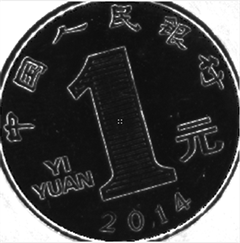Figure 6. Boundary positioning resultFigure 7. Coin sample point image

${r}_{i}=a+d\left(i-1\right)$ (10)

$d=\left(r-a\right)/10$ (11)

5.2. 存储特征模板

$A=\left\{\frac{1,A>\left({A}_{1-}+{A}_{0-}+{A}_{2-}\right)/3}{0,A\le \left({A}_{1-}+{A}_{0-}+{A}_{2-}\right)/3}$ (12)

$135>\left(123+35+245\right)/3=134.44$ (13)

5.3. 数据库建立

5.4. 特征比对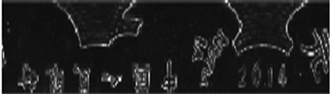Figure 8. Information transformation matrix templateFigure 9. Before binary image pixels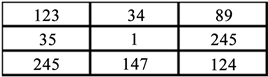Figure 10. A point binarization result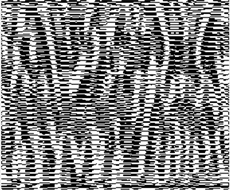Figure 11. Binary feature template

$X=\sum _{i=1}^{10}\sum _{j=1}^{3600}|M\left(i,j\right)-N\left(i,j\right)|$ (14)

6. 识别结果及结果分析

6.1. 识别结果Figure 12. Coin recognition result, (a) One yuan recognition result, (b) One jiao recognition result, (c) Wu jiao recognition result, (d) Non-coin recognition result

6.2. 结果分析

7. 结论

Research of Coin Classification Algorithm Based on Image Processing[J]. 图像与信号处理, 2018, 07(04): 227-235. https://doi.org/10.12677/JISP.2018.74026

1. 1. 关山. 智能小区安防监控系统的设计与实现[D]: [硕士学位论文]. 上海: 上海交通大学, 2015.

2. 2. 龚声蓉, 刘纯平, 王强. 数字图像处理与分析[M]. 北京: 清华大学出社, 2006.

3. 3. 余飞, 刘思宏. 基于特征值的模式匹配算法[J]. 宜宾学院学报, 2014(12).

4. 4. 詹杰斯. 物料状态图像检测法可靠性的DEM研究[D]: [硕士学位论文]. 长沙: 湖南大学, 2014.

5. 5. 徐骏骅. 基于边缘检测与模式识别的车脸识别算法[J]. 控制工程, 2018, 25(2): 357-361.

6. 6. 丁筱玲, 赵强, 李贻斌, 马昕. 基于模板匹配的改进型目标识别算法[J]. 山东大学学报(工学版), 2018, 48(2): 1-7.

7. 7. 衣世东. 基于深度学习的图像识别算法研究[J]. 网络安全技术与应用, 2018(1): 39-41.